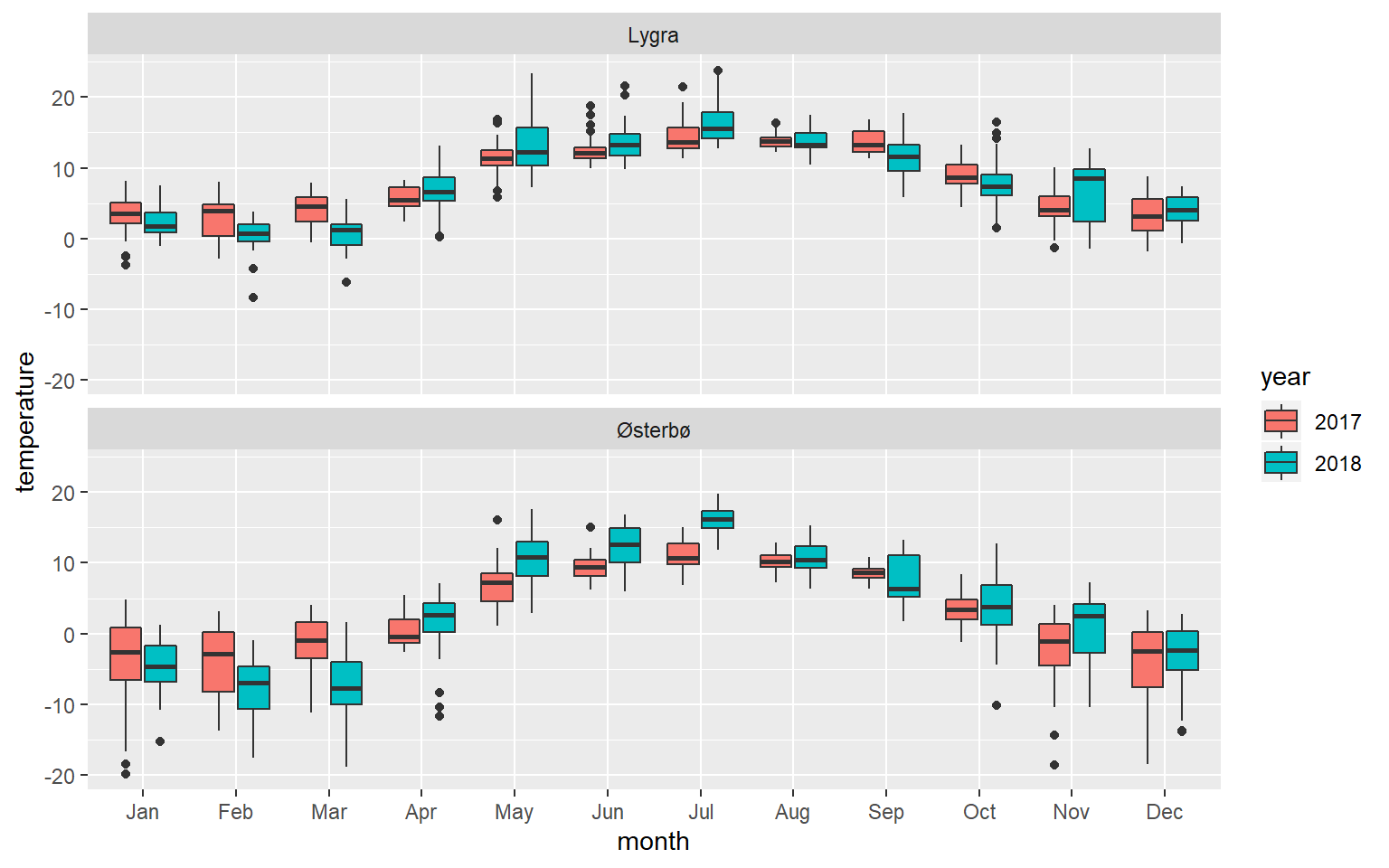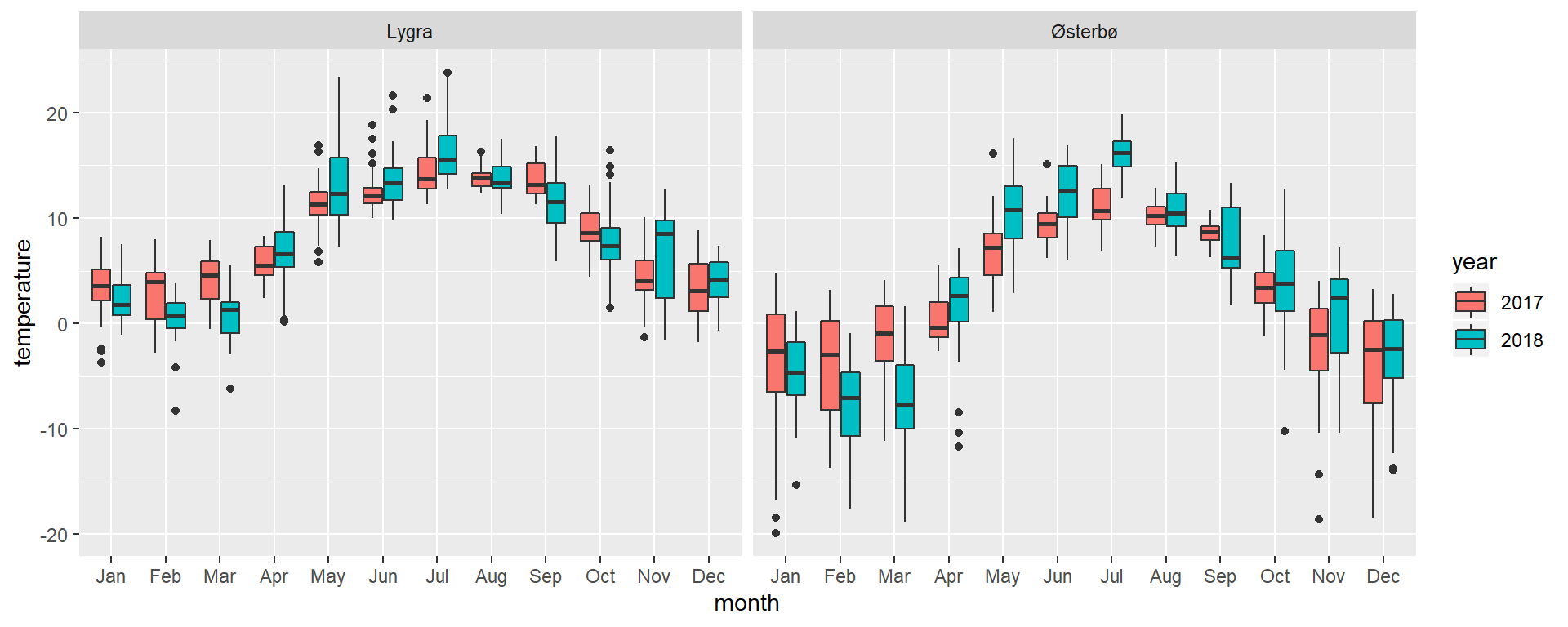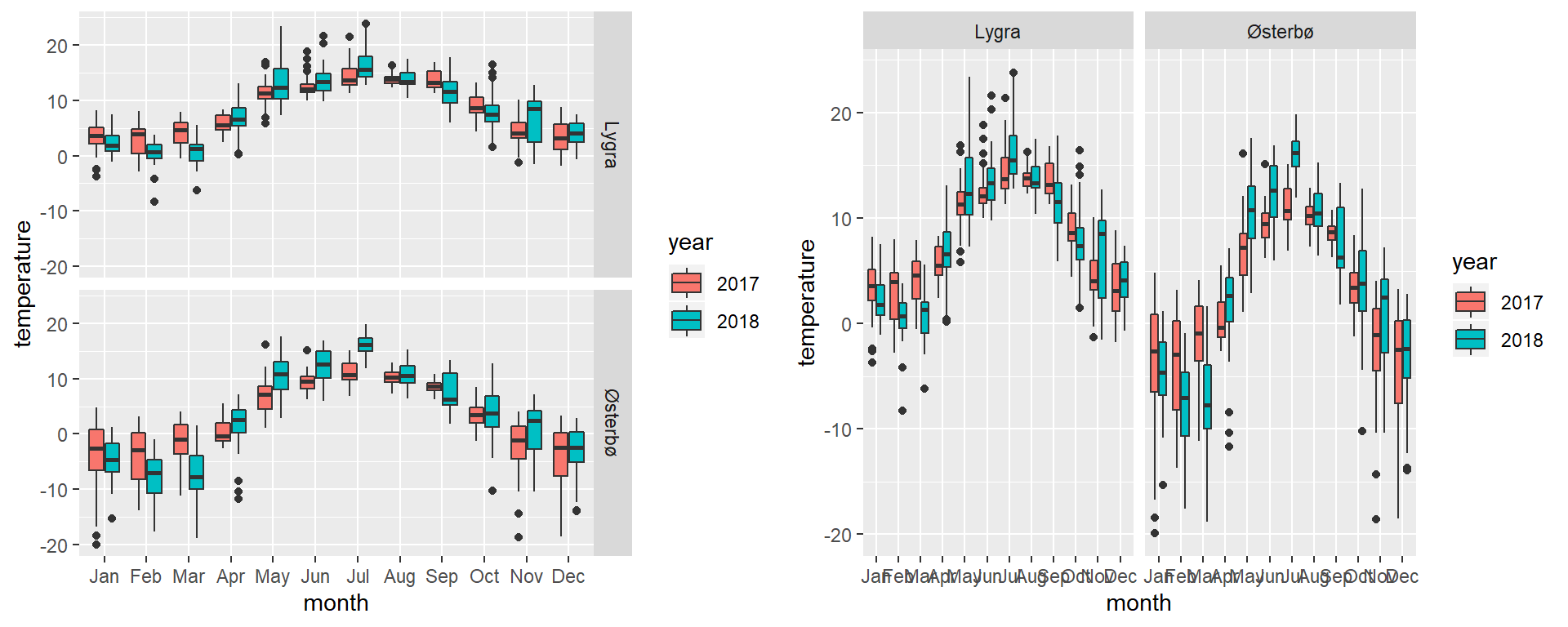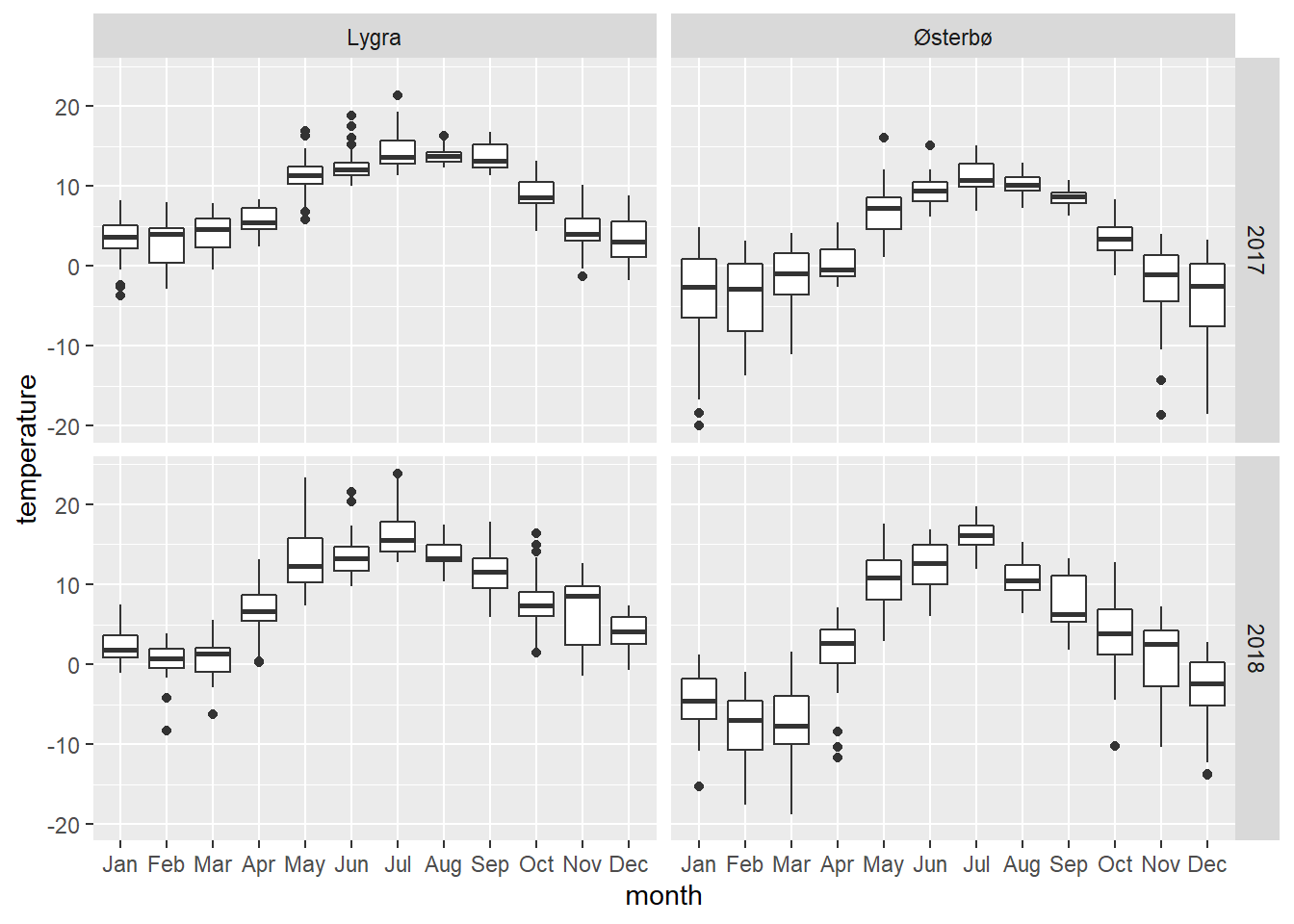In this tutorial, we will see how to make a grid of clustered/grouped boxplots using facet_wrap() and facet_grid(). Such a grid may be useful when your data set contains several categorical predictor variables, and displaying the data in a single graph makes it hardly comprehensible.

If you are not so familiar with boxplots, grouping/clustering data, facet_grid() or facet_wrap(), have a quick look at these pages:

We will plot the temperatures recorded daily in 2018 and 2019 at two Norwegian locations, Lygra and Østerbø in a multiple boxplot. In this plot, each box represents the temperatures for a given month. We will thus have three categorical variables: month, year and location, and one response variable temperature. Here is the code for the dataframe:

# dataframe
df <- data.frame(location, year, month, temperature)
# structure of the dataframe
str(df)
## 'data.frame':    1460 obs. of  4 variables:
##  $location : Factor w/ 2 levels "Lygra","Østerbø": 1 1 1 1 1 1 1 1 1 1 ... ##$ year       : Factor w/ 2 levels "2017","2018": 1 1 1 1 1 1 1 1 1 1 ...
##  $month : Factor w/ 12 levels "Jan","Feb","Mar",..: 1 1 1 1 1 1 1 1 1 1 ... ##$ temperature: num  2.4 0.3 2.8 2.3 -2.4 2.9 2.6 4.6 6.8 5.3 ...

The plan is to make a grid displaying 2 panels, each of which is a clustered boxplot. In these boxplots, the predictor variable month and the response variable temperature shall be plotted on the X- and Y-axis, respectively. Two color-coded boxes shall represent the daily mean temperatures for the recorded months in clusters defined by the variable year. Finally, the grid shall show the two locations on top of each other (two panels displayed in a single column). To obtain this grid, we must:

• map the data for the boxplots by setting up the æsthetics in the following manner: aes(x = month, y = temperature),
• draw the boxes by using geom_boxplot(),
• define the color-coded clusters as a function of the variable year with aes(fill = year)
• create the one-column grid by setting up facet_wrap() like this: facet_wrap(~location, ncol=1).

Here is the code, and the corresponding faceted plot:

ggplot(df, aes(x = month, y = temperature)) +
geom_boxplot(aes(fill = year)) +
facet_wrap(~location, ncol=1)If the plan was to set up a grid with location in a single row instead of a single column, we should have used facet_wrap(~location, nrow=1):

ggplot(df, aes(x = month, y = temperature)) +
geom_boxplot(aes(fill = year)) +
facet_wrap(~location, nrow=1)### facet_wrap() vs facet_grid()

Here above, we have made use of facet_wrap(), but we could have written the code with facet_grid() to achieve the same results. facet_wrap() is easier to use when making a grid based on one variable (here location); on the opposite, facet_grid() requires the use of two variables, unless overridden by:

• rows = vars( ) which shows the levels of the given variable as rows,
• cols = vars( ) which shows the levels of the given variable as columns.
ggplot(df, aes(x = month, y = temperature)) +          # left plot, levels in rows
geom_boxplot(aes(fill = year)) +
facet_grid(rows = vars(location))

ggplot(df, aes(x = month, y = temperature)) +          # right plot, levels in columns
geom_boxplot(aes(fill = year)) +
facet_grid(cols = vars(location))### Improving the look

You may improve the look of a grid by tuning the labels of the grid This is further explained HERE.
Since colors might be important for the interpretation of the data, have a look at this page which shows how to color frames and/or boxes as a function of a variable,, and this page that tells you more about color palettes.

### Alternative plot

This data set may be alternatively plotted in the form of a grid of non-grouped boxplots. HERE is a tutorial for making such a plot.